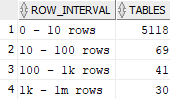## Number of tables by the number of rows in Oracle database

Article for:

If you want to get an overview on how many rows tables in your database hold one way is to count them by row intervals.

Query below returns:

(A) number of all tables accessible to the current user in Oracle database by the number of their rows grouped into predefined intervals

(B) number of all tables in Oracle database by the number of their rows grouped into predefined intervals

Query was executed under the Oracle12c Database version.

## Query

A. Number of tables accessible to the current user

``````select row_interval,
count(*) as tables
from (
select owner,
table_name,
num_rows,
case when num_rows > 1000000000 then '1b rows and more'
when num_rows > 1000000 then '1m - 1b rows'
when num_rows > 1000 then '1k - 1m rows'
when num_rows > 100 then '100 - 1k rows'
when num_rows > 10 then '10 - 100 rows'
else  '0 - 10 rows'
end as row_interval
from sys.all_tables
where owner not in ('ANONYMOUS','CTXSYS','DBSNMP','EXFSYS', 'LBACSYS',
'MDSYS', 'MGMT_VIEW','OLAPSYS','OWBSYS','ORDPLUGINS', 'ORDSYS',
'SI_INFORMTN_SCHEMA','SYS','SYSMAN','SYSTEM', 'TSMSYS','DIP',
'WKPROXY','WMSYS','XDB','APEX_040000', 'APEX_PUBLIC_USER',
'FLOWS_30000','FLOWS_FILES','MDDATA', 'ORACLE_OCM', 'XS\$NULL',
'WK_TEST','WKSYS', 'OUTLN')
)
group by row_interval
order by row_interval;
``````

B. If you have privilege on dba_tables

``````select row_interval,
count(*) as tables
from (
select owner,
table_name,
num_rows,
case when num_rows > 1000000000 then '1b rows and more'
when num_rows > 1000000 then '1m - 1b rows'
when num_rows > 1000 then '1k - 1m rows'
when num_rows > 100 then '100 - 1k rows'
when num_rows > 10 then '10 - 100 rows'
else  '0 - 10 rows' end as row_interval
from sys.dba_tables
where owner not in ('ANONYMOUS','CTXSYS','DBSNMP','EXFSYS', 'LBACSYS',
'MDSYS', 'MGMT_VIEW','OLAPSYS','OWBSYS','ORDPLUGINS', 'ORDSYS',
'SI_INFORMTN_SCHEMA','SYS','SYSMAN','SYSTEM', 'TSMSYS','DIP',
'WKPROXY','WMSYS','XDB','APEX_040000', 'APEX_PUBLIC_USER',
'FLOWS_30000','FLOWS_FILES','MDDATA', 'ORACLE_OCM', 'XS\$NULL',
'WK_TEST','WKSYS', 'OUTLN')
)
group by row_interval
order by row_interval;
``````

## Columns

• row_interval - predefined row count intervals:
• 0 - 10 rows
• 10 - 100 rows
• 100 - 1k rows
• 1k - 1m rows
• 1m - 1b rows
• 1b rows and more
• tables - number of tables that row count falls in that interval

## Rows

• One row represents one interval
• Scope of rows: all row count intervals that appear in the database
• Ordered by row interval

## Sample results